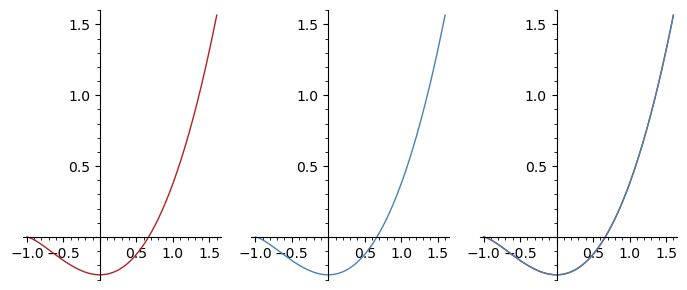# Revision history [back]

To see it by hand, we can use:

• $(x + 1)^{5/2} = (x + 1) (x + 1)^{3/2} = x (x + 1)^{3/2} + (x + 1)^{3/2}$
• $(x + 1)^{3/2} = (x + 1) (x + 1)^{1/2} = x (x + 1)^{1/2} + (x + 1)^{1/2}$

to express both as linear combinations of (x + 1)^(1/2) and x * (x + 1)^(1/2).

To see it with Sage, we can differentiate both and compare them.

Define the function to integrate:

sage: x = var('x')
sage: f = x*sqrt(x + 1)
sage: f
sqrt(x + 1)*x


Integrate with Sage:

sage: F = integral(f, x)
sage: F
2/5*(x + 1)^(5/2) - 2/3*(x + 1)^(3/2)


The primitive you computed (up to a constant):

sage: G = (2/3)*x*(x + 1)^(3/2) - (4/15)*(x + 1)^(5/2)
sage: G
-4/15*(x + 1)^(5/2) + 2/3*(x + 1)^(3/2)*x


To see that they are both correct:

sage: bool(F == G)
True


Or check they have the same derivative:

sage: g = G.diff(x)
sage: g
sqrt(x + 1)*x

sage: ff = F.diff(x)
sage: ff
(x + 1)^(3/2) - sqrt(x + 1)

sage: bool(g == ff)
True


Or more visually, compare the plots of F and G (or f and ff):

sage: pF = plot(F, (-1, 1.6), color='firebrick')
sage: pG = plot(G, (-1, 1.6), color='steelblue')
sage: p = graphics_array([pF, pG, pF + pG])
sage: p.show(figsize=(7, 3))
Launched png viewer for Graphics Array of size 1 x 3To see it by hand, we can use:

• $(x + 1)^{5/2} = (x + 1) (x + 1)^{3/2} = x (x + 1)^{3/2} + (x + 1)^{3/2}$
• $(x + 1)^{3/2} = (x + 1) (x + 1)^{1/2} = x (x + 1)^{1/2} + (x + 1)^{1/2}$

to express both as linear combinations of (x + 1)^(1/2) $(x + 1)^{1/2}$, $x (x + 1)^1/2}$ and x * (x + 1)^(1/2).$x^2 (x + 1)^{1/2}$.

To see it with Sage, we can differentiate both and compare them.

Define the function to integrate:

sage: x = var('x')
sage: f = x*sqrt(x + 1)
sage: f
sqrt(x + 1)*x


Integrate Compute a primitive (aka antiderivative) with Sage:

sage: F = integral(f, x)
sage: F
2/5*(x + 1)^(5/2) - 2/3*(x + 1)^(3/2)


The primitive you computed (up to a constant):

sage: G = (2/3)*x*(x + 1)^(3/2) - (4/15)*(x + 1)^(5/2)
sage: G
-4/15*(x + 1)^(5/2) + 2/3*(x + 1)^(3/2)*x


To see Check that they are both correct:agree:

sage: bool(F == G)
True


Or check Check they have the same derivative:

sage: g = G.diff(x)
sage: g
sqrt(x + 1)*x

sage: ff = F.diff(x)
sage: ff
(x + 1)^(3/2) - sqrt(x + 1)

sage: bool(g == ff)
True


Or more visually, compare the plots of F and G (or f and ff):

sage: pF = plot(F, (-1, 1.6), color='firebrick')
sage: pG = plot(G, (-1, 1.6), color='steelblue')
sage: p = graphics_array([pF, pG, pF + pG])
sage: p.show(figsize=(7, 3))
Launched png viewer for Graphics Array of size 1 x 3To see it by hand, we can use:

• $(x + 1)^{5/2} = (x + 1) (x + 1)^{3/2} = x (x + 1)^{3/2} + (x + 1)^{3/2}$
• $(x + 1)^{3/2} = (x + 1) (x + 1)^{1/2} = x (x + 1)^{1/2} + (x + 1)^{1/2}$

to express both as linear combinations of $(x + 1)^{1/2}$, $x (x + 1)^1/2}$ 1)^{1/2}$and$x^2 (x + 1)^{1/2}\$.

To see it with Sage, we can can

• ask Sage whether the two different expressions in fact agree
• differentiate both and compare them.

them
• plot the functions to visually check whether they agree

Define the function to integrate:

sage: x = var('x')
sage: f = x*sqrt(x + 1)
sage: f
sqrt(x + 1)*x


Compute a primitive (aka antiderivative) with Sage:

sage: F = integral(f, x)
sage: F
2/5*(x + 1)^(5/2) - 2/3*(x + 1)^(3/2)


The primitive you computed (up to a constant):

sage: G = (2/3)*x*(x + 1)^(3/2) - (4/15)*(x + 1)^(5/2)
sage: G
-4/15*(x + 1)^(5/2) + 2/3*(x + 1)^(3/2)*x


Check that they agree:

sage: bool(F == G)
True


Check they have the same derivative:

sage: g = G.diff(x)
sage: g
sqrt(x + 1)*x

sage: ff = F.diff(x)
sage: ff
(x + 1)^(3/2) - sqrt(x + 1)

sage: bool(g == ff)
True


Or more visually, compare the plots of F and G (or f and ff):

sage: pF = plot(F, (-1, 1.6), color='firebrick')
sage: pG = plot(G, (-1, 1.6), color='steelblue')
sage: p = graphics_array([pF, pG, pF + pG])
sage: p.show(figsize=(7, 3))
Launched png viewer for Graphics Array of size 1 x 3